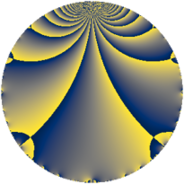# Properties

 Label 2280.2.a.kLevel $2280$ Weight $2$ Character orbit 2280.a Self dual yes Analytic conductor $18.206$ Analytic rank $1$ Dimension $2$ CM no Inner twists $1$

# Related objects

## Newspace parameters

 Level: $$N$$ $$=$$ $$2280 = 2^{3} \cdot 3 \cdot 5 \cdot 19$$ Weight: $$k$$ $$=$$ $$2$$ Character orbit: $$[\chi]$$ $$=$$ 2280.a (trivial)

## Newform invariants

 Self dual: yes Analytic conductor: $$18.2058916609$$ Analytic rank: $$1$$ Dimension: $$2$$ Coefficient field: $$\Q(\sqrt{2})$$ Defining polynomial: $$x^{2} - 2$$ Coefficient ring: $$\Z[a_1, \ldots, a_{7}]$$ Coefficient ring index: $$1$$ Twist minimal: yes Fricke sign: $$1$$ Sato-Tate group: $\mathrm{SU}(2)$

## $q$-expansion

Coefficients of the $$q$$-expansion are expressed in terms of $$\beta = \sqrt{2}$$. We also show the integral $$q$$-expansion of the trace form.

 $$f(q)$$ $$=$$ $$q - q^{3} - q^{5} + \beta q^{7} + q^{9} +O(q^{10})$$ $$q - q^{3} - q^{5} + \beta q^{7} + q^{9} + ( -2 + \beta ) q^{11} + ( 2 - \beta ) q^{13} + q^{15} -2 \beta q^{17} + q^{19} -\beta q^{21} + ( -2 - 2 \beta ) q^{23} + q^{25} - q^{27} -5 \beta q^{29} + ( -2 - 2 \beta ) q^{31} + ( 2 - \beta ) q^{33} -\beta q^{35} + ( 2 + 3 \beta ) q^{37} + ( -2 + \beta ) q^{39} + 7 \beta q^{41} + ( 4 + 5 \beta ) q^{43} - q^{45} + ( -6 + 2 \beta ) q^{47} -5 q^{49} + 2 \beta q^{51} + 6 \beta q^{53} + ( 2 - \beta ) q^{55} - q^{57} + ( -4 + 2 \beta ) q^{59} + ( 4 - 4 \beta ) q^{61} + \beta q^{63} + ( -2 + \beta ) q^{65} -8 \beta q^{67} + ( 2 + 2 \beta ) q^{69} + ( -12 - 2 \beta ) q^{71} + ( -6 - 4 \beta ) q^{73} - q^{75} + ( 2 - 2 \beta ) q^{77} + ( -8 - 4 \beta ) q^{79} + q^{81} + ( -2 - 4 \beta ) q^{83} + 2 \beta q^{85} + 5 \beta q^{87} + ( -8 - 3 \beta ) q^{89} + ( -2 + 2 \beta ) q^{91} + ( 2 + 2 \beta ) q^{93} - q^{95} + ( 2 + 7 \beta ) q^{97} + ( -2 + \beta ) q^{99} +O(q^{100})$$ $$\operatorname{Tr}(f)(q)$$ $$=$$ $$2 q - 2 q^{3} - 2 q^{5} + 2 q^{9} + O(q^{10})$$ $$2 q - 2 q^{3} - 2 q^{5} + 2 q^{9} - 4 q^{11} + 4 q^{13} + 2 q^{15} + 2 q^{19} - 4 q^{23} + 2 q^{25} - 2 q^{27} - 4 q^{31} + 4 q^{33} + 4 q^{37} - 4 q^{39} + 8 q^{43} - 2 q^{45} - 12 q^{47} - 10 q^{49} + 4 q^{55} - 2 q^{57} - 8 q^{59} + 8 q^{61} - 4 q^{65} + 4 q^{69} - 24 q^{71} - 12 q^{73} - 2 q^{75} + 4 q^{77} - 16 q^{79} + 2 q^{81} - 4 q^{83} - 16 q^{89} - 4 q^{91} + 4 q^{93} - 2 q^{95} + 4 q^{97} - 4 q^{99} + O(q^{100})$$

## Embeddings

For each embedding $$\iota_m$$ of the coefficient field, the values $$\iota_m(a_n)$$ are shown below.

For more information on an embedded modular form you can click on its label.

Label $$\iota_m(\nu)$$ $$a_{2}$$ $$a_{3}$$ $$a_{4}$$ $$a_{5}$$ $$a_{6}$$ $$a_{7}$$ $$a_{8}$$ $$a_{9}$$ $$a_{10}$$
1.1
 −1.41421 1.41421
0 −1.00000 0 −1.00000 0 −1.41421 0 1.00000 0
1.2 0 −1.00000 0 −1.00000 0 1.41421 0 1.00000 0
 $$n$$: e.g. 2-40 or 990-1000 Significant digits: Format: Complex embeddings Normalized embeddings Satake parameters Satake angles

## Atkin-Lehner signs

$$p$$ Sign
$$2$$ $$-1$$
$$3$$ $$1$$
$$5$$ $$1$$
$$19$$ $$-1$$

## Inner twists

This newform does not admit any (nontrivial) inner twists.

## Twists

By twisting character orbit
Char Parity Ord Mult Type Twist Min Dim
1.a even 1 1 trivial 2280.2.a.k 2
3.b odd 2 1 6840.2.a.bb 2
4.b odd 2 1 4560.2.a.bn 2

By twisted newform orbit
Twist Min Dim Char Parity Ord Mult Type
2280.2.a.k 2 1.a even 1 1 trivial
4560.2.a.bn 2 4.b odd 2 1
6840.2.a.bb 2 3.b odd 2 1

## Hecke kernels

This newform subspace can be constructed as the intersection of the kernels of the following linear operators acting on $$S_{2}^{\mathrm{new}}(\Gamma_0(2280))$$:

 $$T_{7}^{2} - 2$$ $$T_{11}^{2} + 4 T_{11} + 2$$ $$T_{13}^{2} - 4 T_{13} + 2$$

## Hecke characteristic polynomials

$p$ $F_p(T)$
$2$ $$T^{2}$$
$3$ $$( 1 + T )^{2}$$
$5$ $$( 1 + T )^{2}$$
$7$ $$-2 + T^{2}$$
$11$ $$2 + 4 T + T^{2}$$
$13$ $$2 - 4 T + T^{2}$$
$17$ $$-8 + T^{2}$$
$19$ $$( -1 + T )^{2}$$
$23$ $$-4 + 4 T + T^{2}$$
$29$ $$-50 + T^{2}$$
$31$ $$-4 + 4 T + T^{2}$$
$37$ $$-14 - 4 T + T^{2}$$
$41$ $$-98 + T^{2}$$
$43$ $$-34 - 8 T + T^{2}$$
$47$ $$28 + 12 T + T^{2}$$
$53$ $$-72 + T^{2}$$
$59$ $$8 + 8 T + T^{2}$$
$61$ $$-16 - 8 T + T^{2}$$
$67$ $$-128 + T^{2}$$
$71$ $$136 + 24 T + T^{2}$$
$73$ $$4 + 12 T + T^{2}$$
$79$ $$32 + 16 T + T^{2}$$
$83$ $$-28 + 4 T + T^{2}$$
$89$ $$46 + 16 T + T^{2}$$
$97$ $$-94 - 4 T + T^{2}$$